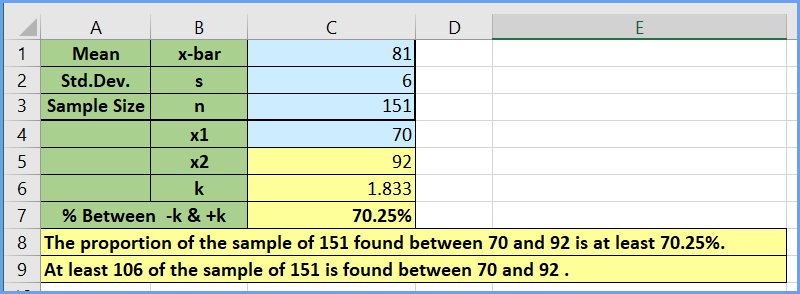# Chebyshev’s Theorem Excel Calculator

## Chebyshev's Excel Calculator

Chebyshev’s Theorem can be used for any type of distribution, but if the problem says the distribution is “bell shaped,” use the Empirical Rule unless the problem specifically says to use Chebyshev’s.

Note: an alternative spelling is Chebychev.

Chebyshev’s only applies to problems where the K is > 1. In our MSL Larson 233, K is an integer: 2, 3, or 4. But some authors say “any number >1” so you can use the theorem for other z-scores. See                 tab for example of this type of problem

Remember, Chebyshev’s Theorem says the portion of any data set lying within k standard deviations of the mean is at least 1−(1/k^2).

Image credit : https://bit.ly/3rWgGMr

Problem Type 1 Procedure:

1. Enter the mean (x-bar), the standard deviation, and the sample size as stated in the problem in the blue cells. If n is not given, leave the current value and ignore the statement in row 9.
2. Enter the lower x value stated in the problem in cell C4. Because intro stats problems will usually give you matched pairs of lower and upper values, the upper value of x should appear in cell C5. If your upper value is not shown in cell C5, check with your instructor for guidance.
3. k is shown in cell C5 and the proportion between -k and +k is shown in cell C6. Conclusion statements are in rows 8 and 9.
4. Remember, Chebyshev’s Theorem says “at least” that percentage between the lower and upper limits. And “at least” that number between.Problem Type 2

In this type of problem, you are given the mean and standard deviation. You may also be given the sample size. The problem will often ask you to find the percentage of data between upper and lower values corresponding to 2, 3, or 4 standard deviations. Sometimes you will also be asked to find the Number of data points between.

Procedure

1.  Enter the mean (x-bar) and the standard deviation as stated in the problem in the blue cells. If a sample size, n, is stated, enter that. If no n is stated, leave the current value.
2. Find the lower and upper values stated in the problem in the yellow B and C columns. Because Chebyshev’s only applies to integer values of k, intro stats problems will usually give you matched pairs of lower and upper values.
3. Find your pair of values and read the % between and number between in columns E and F on that row.
• 3a. If the problem gives a value for k, the number of standard deviations, find it in columns A and look on that row.
• 3b. If you do not know the true sample size, n, do not use the “Number Between” values as they are meaningless for your problem.
1. Remember, Chebyshev’s Theorem says “at least” that percentage between the lower and upper limits. And “at least” that number between.
2. If the problem upper and lower values do not match those in the table, double check you have entered the mean and standard deviation correctly. Then double-check the lower and upper values in the problem statement. If you do not see an obvious error, contact your instructor.